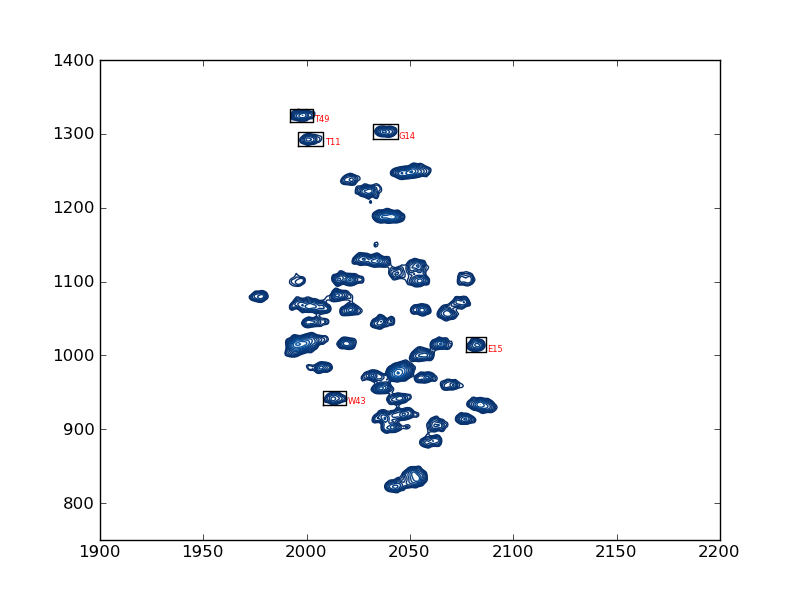# plotting example: plot_2d_assignments

This example shows how to use nmrglue and matplotlib to create figures for examining data or publication. In this example the assignments used in integration example: integrate_2d are graphically examined. A contour plot of the spectrum with the boxes and assignments is created. To examine the box limit more closely see plotting example: plot_2d_boxes.

```#! /usr/bin/env python
# Create a contour plots of a spectrum with each peak in limits.in labeled

import nmrglue as ng
import numpy as np
import matplotlib.pyplot as plt
import matplotlib.cm

# plot parameters
cmap = matplotlib.cm.Blues_r    # contour map (colors to use for contours)
contour_start = 30000           # contour level start value
contour_num = 20                # number of contour levels
contour_factor = 1.20           # scaling factor between contour levels
textsize = 6                    # text size of labels

# calculate contour levels
cl = contour_start * contour_factor ** np.arange(contour_num)

# read in the data from a NMRPipe file

# read in the integration limits
peak_list = np.recfromtxt("limits.in")

# create the figure
fig = plt.figure()

# plot the contours
ax.contour(data, cl, cmap=cmap,
extent=(0, data.shape - 1, 0, data.shape - 1))

# loop over the peaks
for name, x0, y0, x1, y1 in peak_list:

if x0 > x1:
x0, x1 = x1, x0
if y0 > y1:
y0, y1 = y1, y0

# plot a box around each peak and label
ax.plot([x0, x1, x1, x0, x0], [y0, y0, y1, y1, y0], 'k')
ax.text(x1 + 1, y0, name, size=textsize, color='r')

# set limits
ax.set_xlim(1900, 2200)
ax.set_ylim(750, 1400)

# save the figure
fig.savefig("assignments.png")
```
```#Peak   X0      Y0      X1      Y1
# Peak defines 15N resonance in 2D NCO spectra.
# Limits are in term of points from 0 to length-1.
# These can determined from nmrDraw by subtracting 1 from the X and Y
# values reported.
#Peak   X0      Y0      X1      Y1
T49     1992    1334    2003    1316
T11     1996    1302    2008    1284
# comments can appear anywhere in this file just start the line with #
G14     2032    1314    2044    1293
E15     2077    1025    2087    1004
W43     2008    952     2019    933
```

Result: